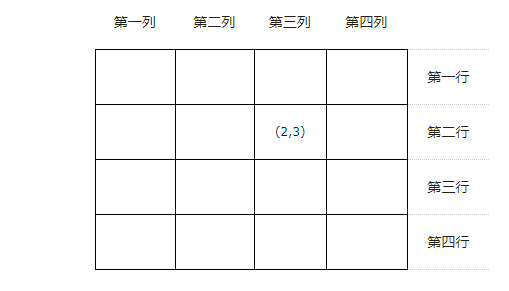# #57. 同行列对角线的格子

#### 题目描述(2,1) (2,2) (2,3) (2,4) //同一行上格子的位置

(1,3) (2,3) (3,3) (4,3) //同一列上格子的位置

(1,2) (2,3) (3,4) //左上到右下对角线上的格子的位置

(4,1) (3,2) (2,3) (1,4) //左下到右上对角线上的格子的位置

#### 样例输入

4 2 3


#### 样例输出

(2,1) (2,2) (2,3) (2,4)
(1,3) (2,3) (3,3) (4,3)
(1,2) (2,3) (3,4)
(4,1) (3,2) (2,3) (1,4)